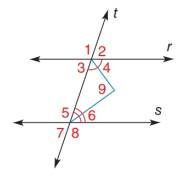Chapter 2.1, Problem 7E### Elementary Geometry for College St...

6th Edition
Daniel C. Alexander + 1 other
ISBN: 9781285195698

#### Solutions

Chapter
Section### Elementary Geometry for College St...

6th Edition
Daniel C. Alexander + 1 other
ISBN: 9781285195698
Textbook Problem
1 views

# Use drawings, as needed, to answer each question.Suppose that r ∥ s . Both interior angles ( ∠ 4 and ∠ 6 ) have been bisected. Using intuition, what appears to be true of ∠ 9 formed by the bisectors?To determine

To find:

The value of m9.

Explanation

Given:

The given figure is,

rs and both interior angles (4 and 6) have been bisected.

Definition:

The pairs of angles on one side of the transversal but inside the two lines are called consecutive interior Angles. Sum of measures of consecutive interior angles is 180°.

Calculation:

Consider the given figure,

4and6 are forming consecutive interior angles. Sum of measures of consecutive interior angles is 180°.

m6+m4=180°

Since 4and6 are bisected.

12(m6+m4)=12×180°12m6+12m4=90°

### Still sussing out bartleby?

Check out a sample textbook solution.

See a sample solution

#### The Solution to Your Study Problems

Bartleby provides explanations to thousands of textbook problems written by our experts, many with advanced degrees!

Get Started

#### f(x) = 3x3 x2 + 10; limxf(x); and limxf(x) x 1 5 10 100 1000 f(x) x 1 5 10 100 1000 f(x)

Applied Calculus for the Managerial, Life, and Social Sciences: A Brief Approach

#### Place the following set of n = 20 scores in a frequency distribution table.

Essentials of Statistics for The Behavioral Sciences (MindTap Course List)

#### Show that if x+312, then |4x + 13| 3.

Single Variable Calculus: Early Transcendentals, Volume I

#### True or False: converges.

Study Guide for Stewart's Multivariable Calculus, 8th

#### limxx3ex/2

Calculus: Early Transcendental Functions (MindTap Course List)

#### What is a ceiling effect, and how can it be a problem?

Research Methods for the Behavioral Sciences (MindTap Course List)